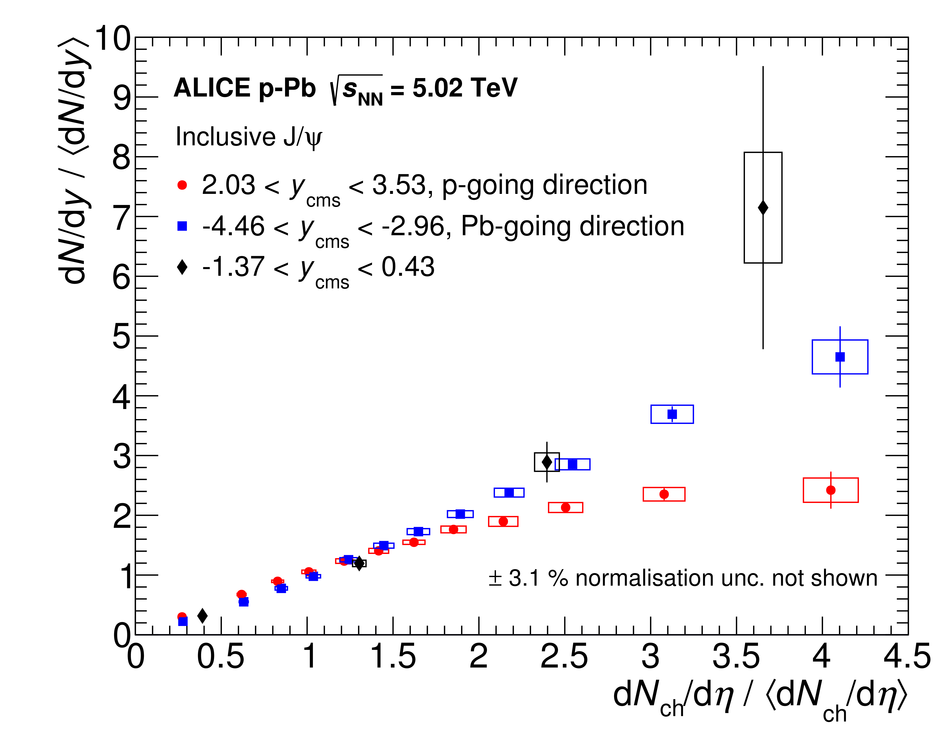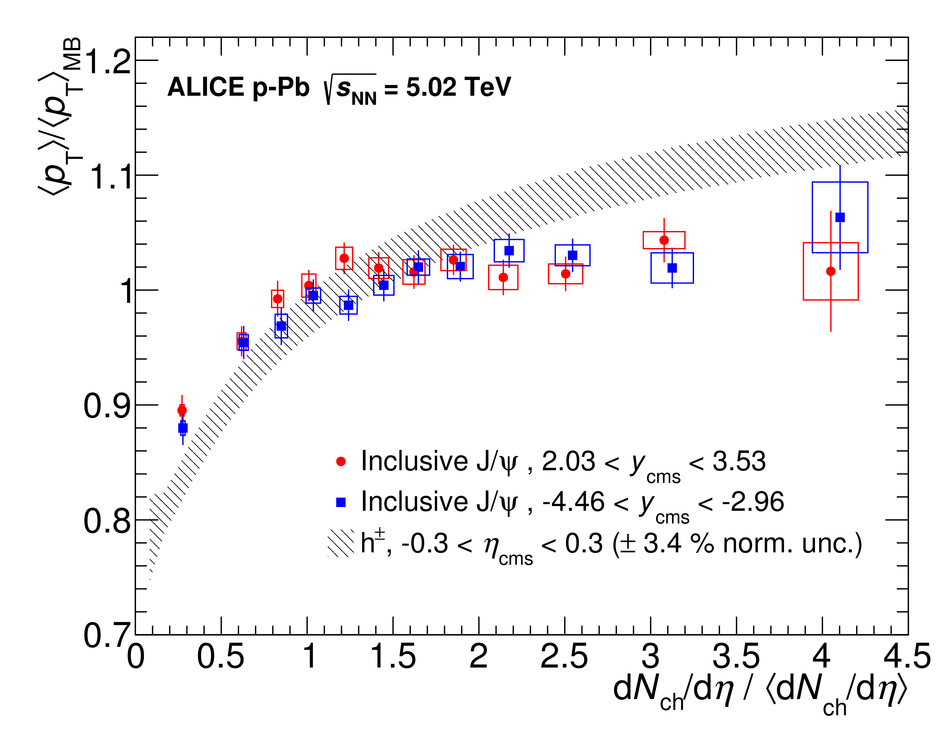# J/$ψ$ production as a function of charged-particle pseudorapidity density in p-Pb collisions at $\sqrt{s_{\rm NN}} = 5.02$ TeV

We report measurements of the inclusive J/$\psi$ yield and average transverse momentum as a function of charged-particle pseudorapidity density ${\rm d}N_{\rm ch}/{\rm d}\eta$ in p-Pb collisions at $\sqrt{s_{\rm NN}} = 5.02$ TeV with ALICE at the LHC. The observables are normalised to their corresponding averages in non-single diffractive events. An increase of the normalised J/$\psi$ yield with normalised ${\rm d}N_{\rm ch}/{\rm d}\eta$ is observed at mid-rapidity and backward rapidity, similar to that found earlier in pp collisions. At forward rapidity, a saturation of the relative yield is observed for high charged-particle multiplicities. The normalised average transverse momentum at forward and backward rapidity increases with multiplicity at low multiplicities and saturates beyond moderate multiplicities. In addition, the forward-to-backward nuclear modification factor ratio is also reported, showing an increasing suppression of J/$\psi$ production at forward rapidity with respect to backward rapidity for increasing charged-particle multiplicity.

Figures

## Figure 1

 Opposite-sign invariant mass distributions of selected muon (left panel, for the forward rapidity) and electron (right panel) pairs, for selected multiplicity bins. In the left panel, the distributions are corrected for \acef. The curves show the fit functions for signal, background and combined signal and background (see text for details). In the right panel, the background is evaluated with the event-mixing technique, the overlaid signal is obtained from Monte Carlo (see text for details).## Figure 2

 Average transverse momentum of opposite-sign muon pairs as a function of the invariant mass at forward rapidity, for two multiplicity bins. The curves are fits of the background and combined signal and background (see text).## Figure 3

 Relative yield of inclusive \jpsi mesons as a function of relative charged-particle pseudorapidity density for three rapidity regions The error bars show the statistical uncertainties, the boxes the systematic ones## Figure 4

 Relative yield of inclusive \jpsi mesons as a function of relative charged-particle pseudorapidity density in comparison to D mesons (average of D$^0$, D$^+$, and D$^{*+}$ species), for the \pt ~interval 2-4 \gevc ~ The error bars show the statistical uncertainties, the boxes the systematic ones (for the D mesons, additional systematic uncertainties due to the b feed-down contributions and the event normalisation are not shown)## Figure 5

 $R_{\mathrm{FB}}$ of inclusive \jpsi in p-Pb collisions at $\sqrt{s_{\mathrm{NN}}} =$ 5.02 TeV as a function of relative charged-particle pseudorapidity density. The red box around unity represents the global uncertainty The error bars show the statistical uncertainties, the boxes the systematic ones## Figure 6

 Relative \mpt ~of \jpsi mesons for backward and forward rapidity as a function of the relative charged-particle pseudorapidity density. The bars show the statistical uncertainties, the boxes the systematic ones. The data for charged particles (h$^\pm$) are included for comparison. The latter are for $|\etacms|< 0.3$ and with \pt ~in the range 0.15 to 10 \gevc ~and have an additional normalisation uncertainty of 3.4\%